table.module-1{width:53.4%;padding:0}table div table+table div table{width:53.4%;float:none;margin-left:auto;margin-right:auto;padding:0}table div table+table div table a{border:0 none;text-decoration:none}table div table+table div table img{width:100%!important;border:0 none;text-decoration:none}table div table+table div table td{width:100%;padding:0}/* styles */

## Helllooooo everyone! Your bear Jigsaw here…..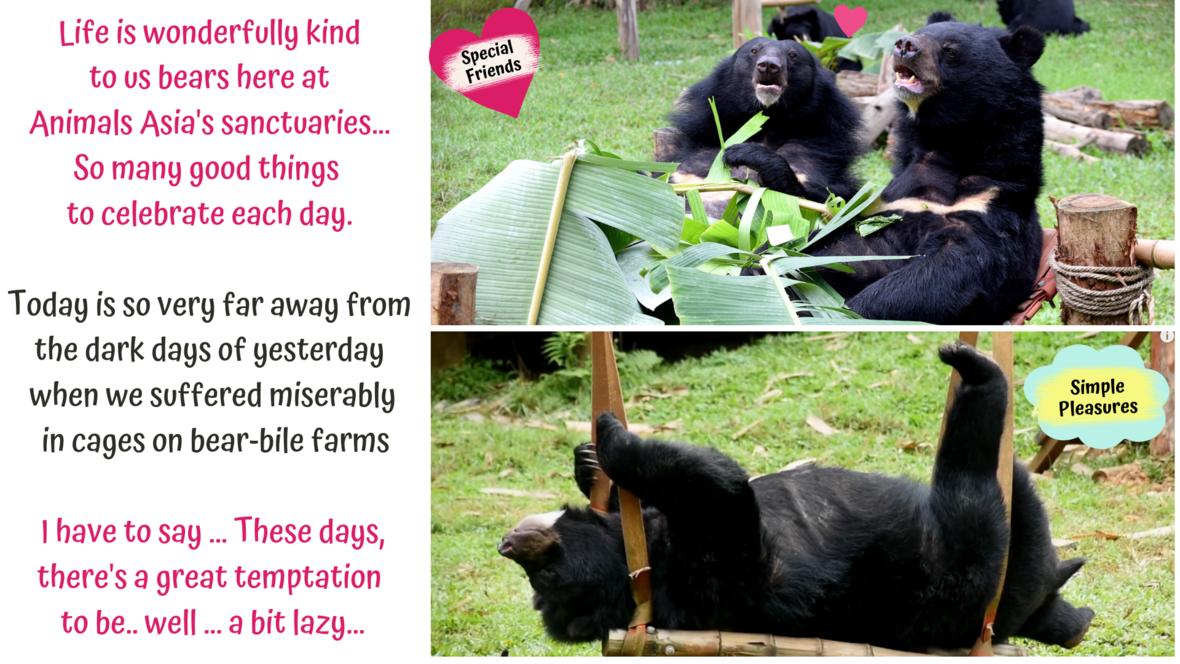table div table+table+table+table+table div table{width:100%;padding:0}table div table+table+table+table+table div table img{width:96.23%;padding:0;float:none}table div table+table+table+table+table div table td{width:100%;padding:0 1.88% 18px}/* styles */## But there's lots going on! *More lucky bears joining us *History being made *Awards being won *Precious gifts being received. So let's get going with the news!

 table div table+table+table+table+table+table+table div table{width:100%;padding:0}table div table+table+table+table+table+table+table div table img{width:96.23%;padding:0;float:none}table div table+table+table+table+table+table+table div table td{width:100%;padding:0 1.88% 18px}/* styles */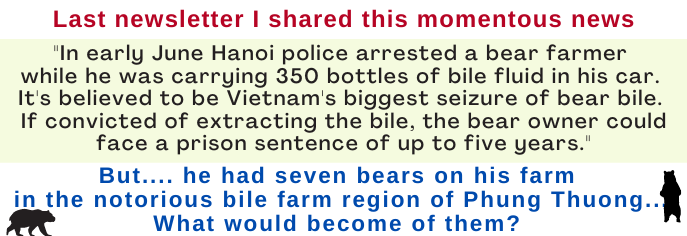table div table+table+table+table+table+table+table+table+table div table{width:100%;padding:0}table div table+table+table+table+table+table+table+table+table div table img{width:96.23%;padding:0;float:none}table div table+table+table+table+table+table+table+table+table div table td{width:100%;padding:0 1.88% 18px}/* styles */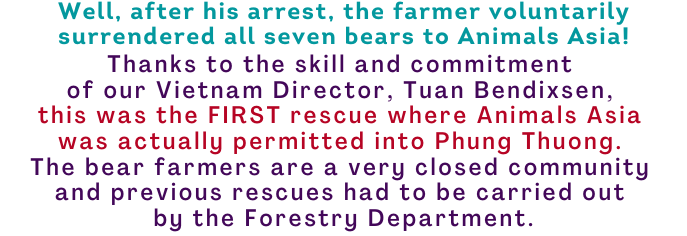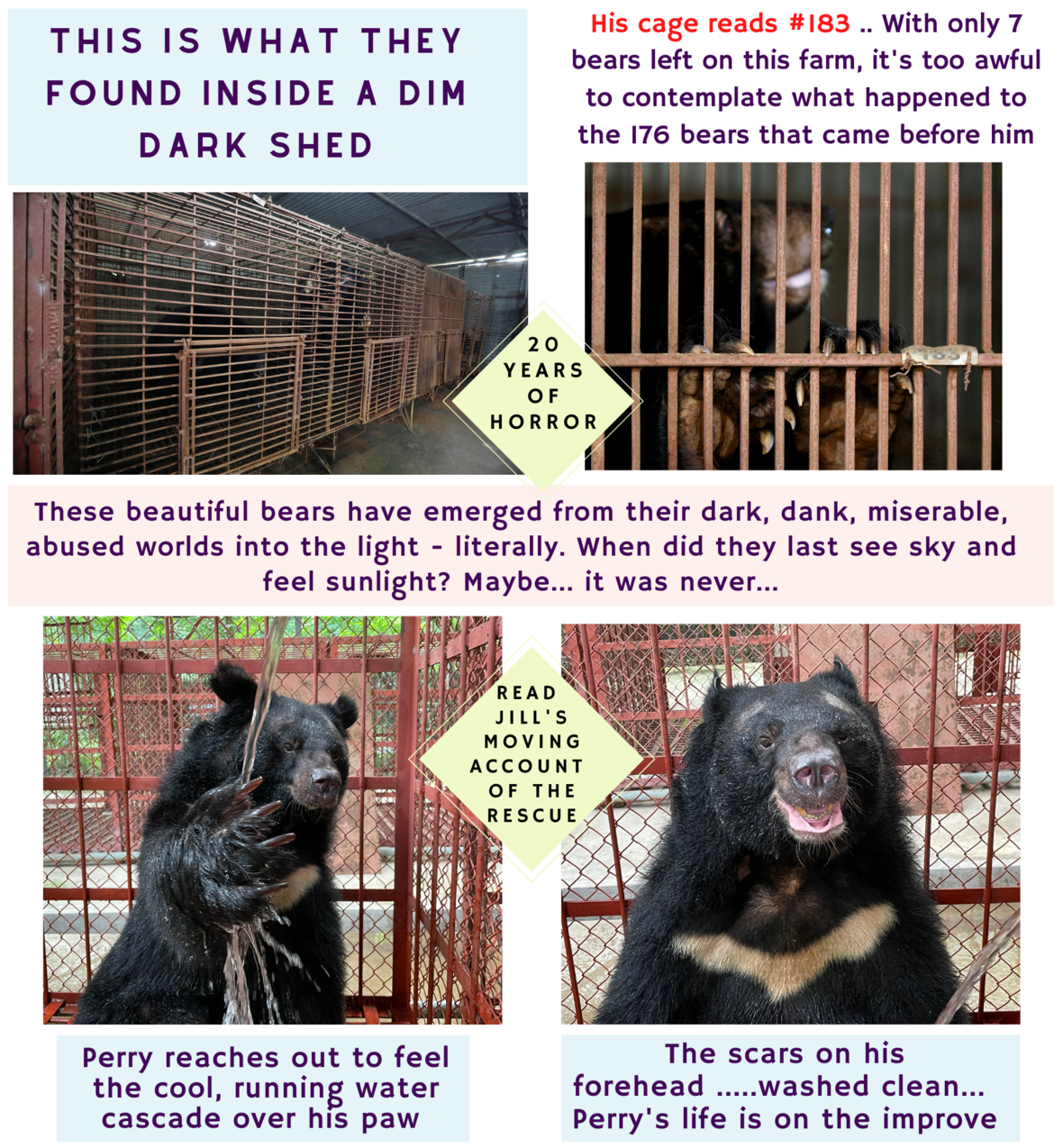JILL REFLECTS ON THE LIFE OF THE RESCUED "LOTUS FLOWER" BEARS
 table div table+table+table+table+table+table+table+table+table+table+table+table div table{width:100%;padding:0}table div table+table+table+table+table+table+table+table+table+table+table+table div table img{width:96.23%;padding:0;float:none}table div table+table+table+table+table+table+table+table+table+table+table+table div table td{width:100%;padding:0 1.88% 18px}/* styles */## Already (happily) bulging with bears we were asked to rescue two more in September! Well we just have to offer them care and kindness after 17 truly awful years in cages .. and that's why YOUR help is so appreciated.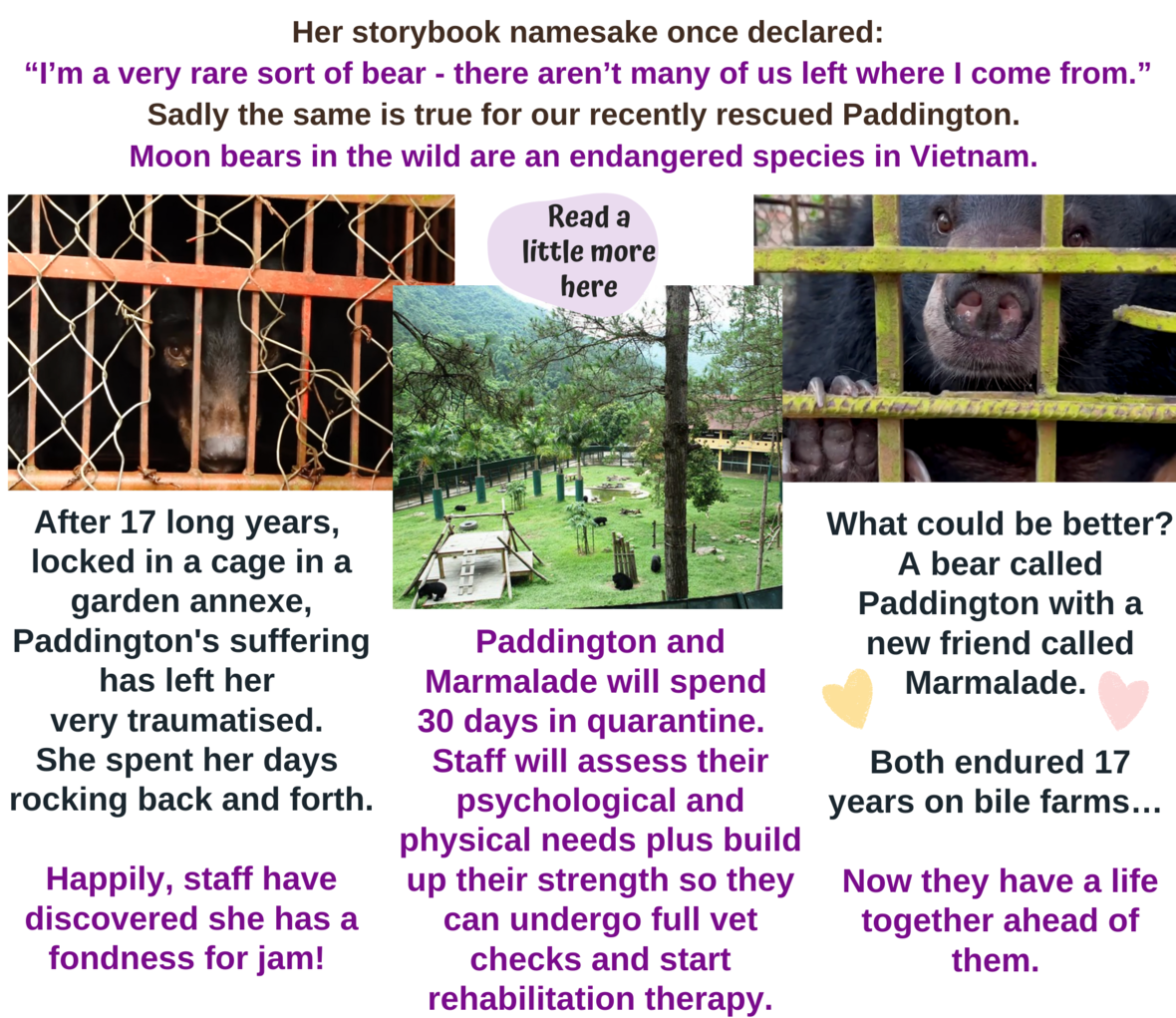WHY THE NAME PADDINGTON? FIND OUT HERE
 table div table+table+table+table+table+table+table+table+table+table+table+table+table+table+table div table{width:100%;padding:0}table div table+table+table+table+table+table+table+table+table+table+table+table+table+table+table div table img{width:96.23%;padding:0;float:none}table div table+table+table+table+table+table+table+table+table+table+table+table+table+table+table div table td{width:100%;padding:0 1.88% 18px}/* styles */## Now, I thought my name was cool but Beserk the bear wins, paws down! Beserk is one of those bears who adapted quickly and enthusiastically to his new life. He's full of fun & happiness – after only 4 months!

 table div table+table+table+table+table+table+table+table+table+table+table+table+table+table+table+table+table div table td,table.module-16{width:100%;padding:0}table div table+table+table+table+table+table+table+table+table+table+table+table+table+table+table+table+table div table{width:100%;float:none;margin-left:auto;margin-right:auto;padding:0}table div table+table+table+table+table+table+table+table+table+table+table+table+table+table+table+table+table div table a{border:0 none;text-decoration:none}table div table+table+table+table+table+table+table+table+table+table+table+table+table+table+table+table+table div table img{width:100%!important;border:0 none;text-decoration:none}/* styles */
 table div table+table+table+table+table+table+table+table+table+table+table+table+table+table+table+table+table+table div table{width:100%;padding:0}table div table+table+table+table+table+table+table+table+table+table+table+table+table+table+table+table+table+table div table img{width:96.23%;padding:0;float:none}table div table+table+table+table+table+table+table+table+table+table+table+table+table+table+table+table+table+table div table td{width:100%;padding:0 1.88% 18px}/* styles */## Of course not everyone turns into Beserk the Bear !! Some bears go more gently and quietly about their sanctuary lives, grateful for the peace it brings them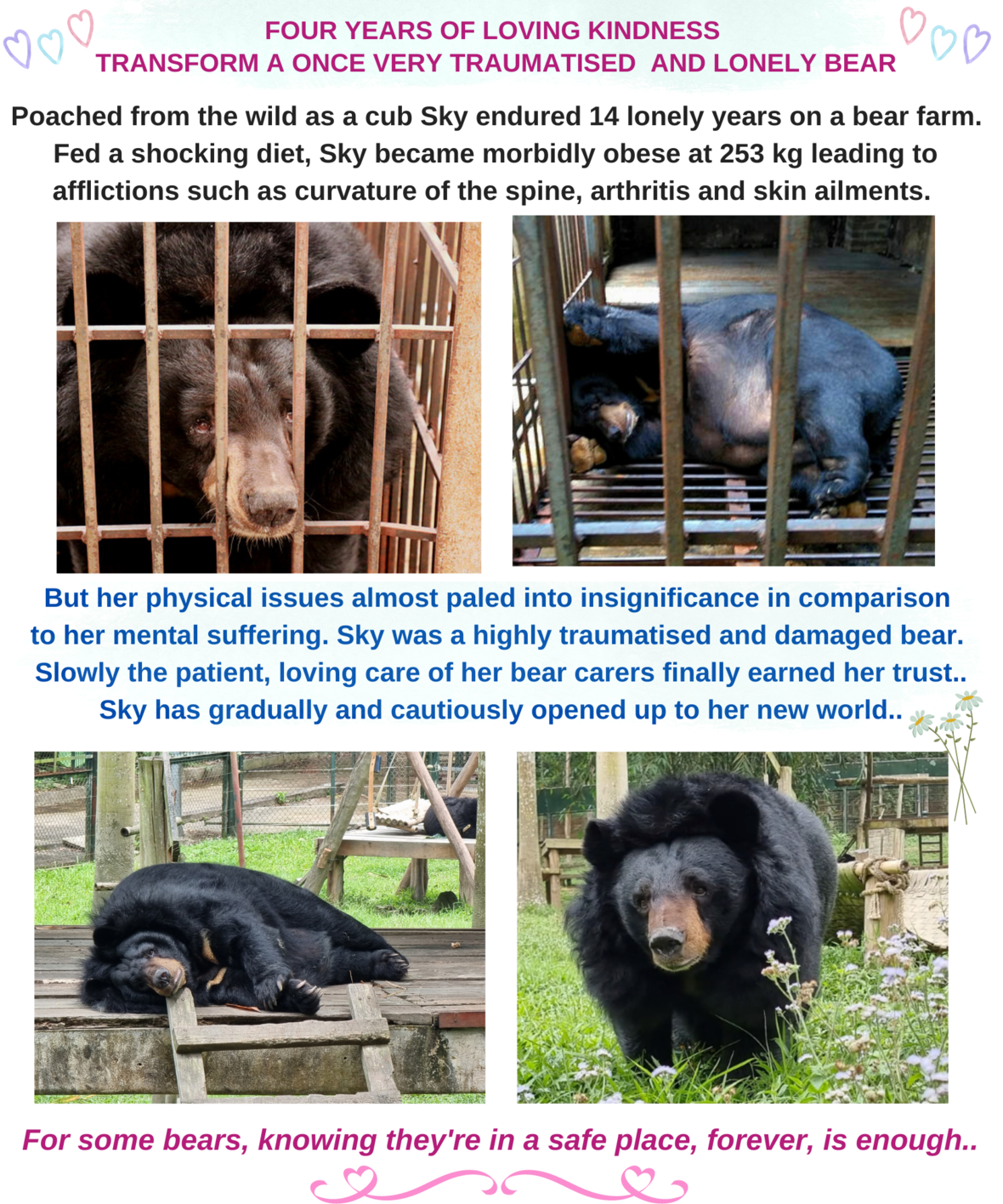table div table+table+table+table+table+table+table+table+table+table+table+table+table+table+table+table+table+table+table+table+table div table{width:100%;padding:0}table div table+table+table+table+table+table+table+table+table+table+table+table+table+table+table+table+table+table+table+table+table div table img{width:96.23%;padding:0;float:none}table div table+table+table+table+table+table+table+table+table+table+table+table+table+table+table+table+table+table+table+table+table div table td{width:100%;padding:0 1.88% 18px}/* styles */## Some very exciting news for "team" Adopt A Moon Bear! Congratulations to YOU and Sal for winning one of Animals Asia's prestigious awards in 2021!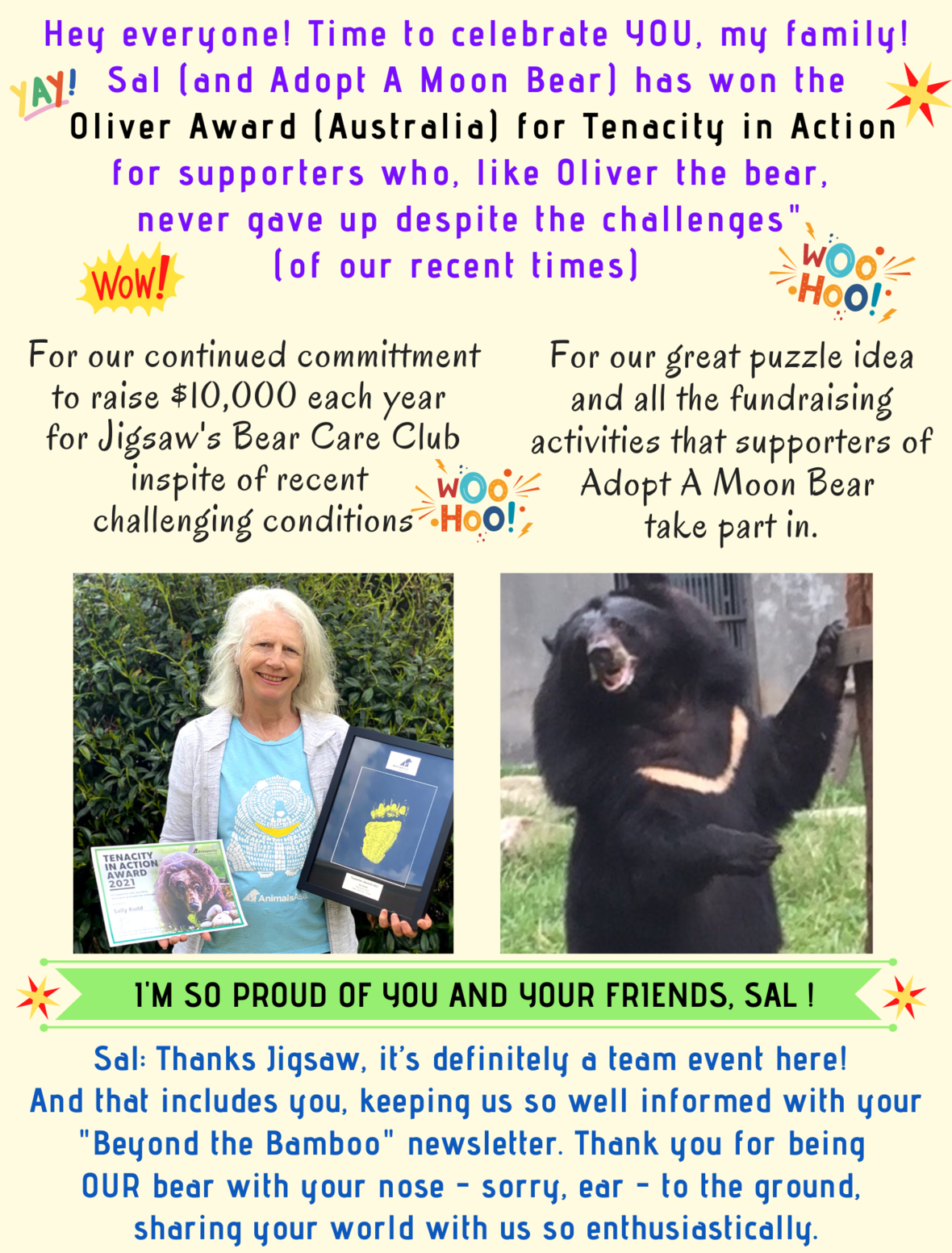## Sal would like to say a few words as an Oliver Award has special meaning for her…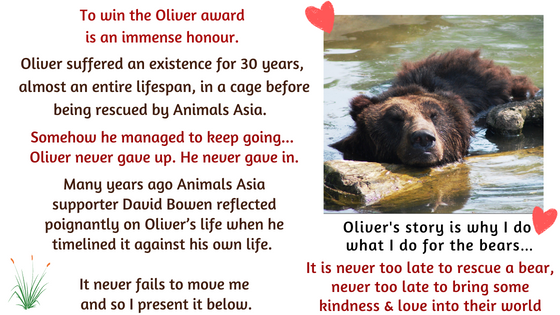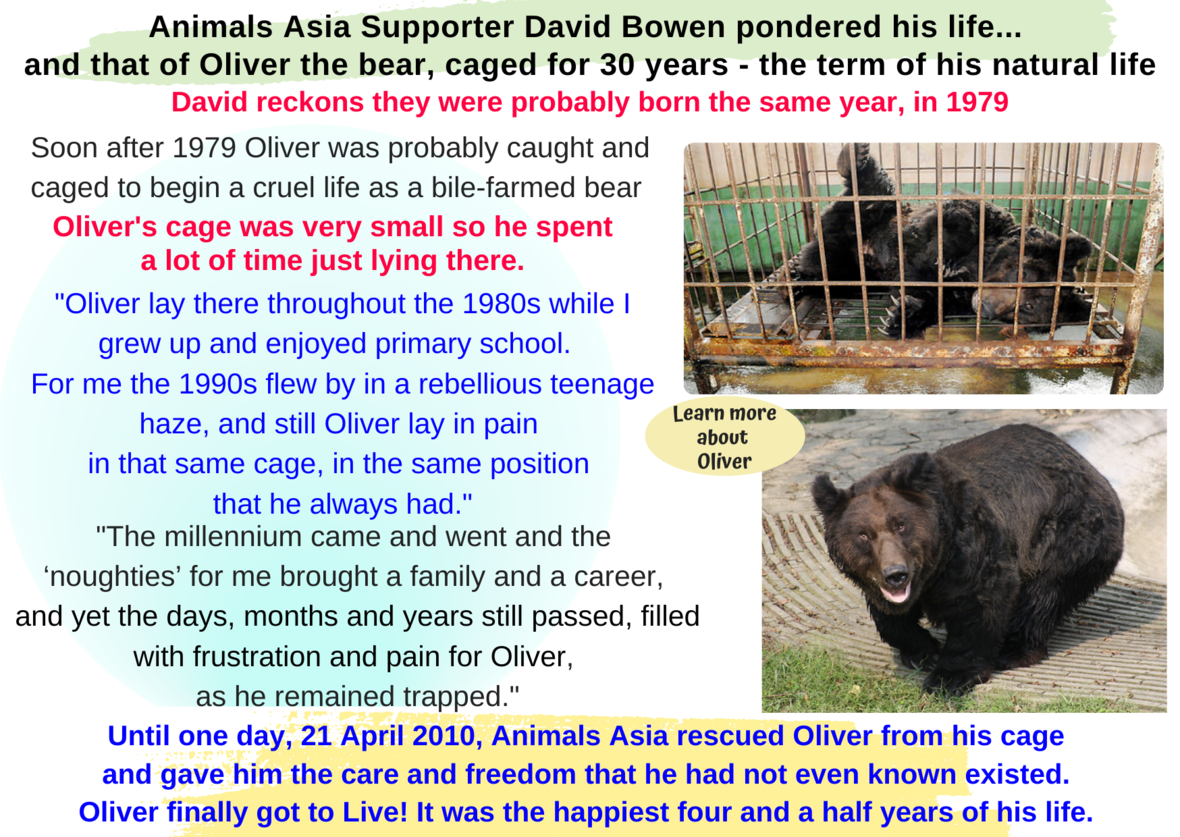OLIVER, A BEAR WHO INSPIRED A MOVEMENT

## Thanks Sal. I'm hoping to live to 30 and am so very grateful I only suffered 5 years on a bile farm....

 table div table+table+table+table+table+table+table+table+table+table+table+table+table+table+table+table+table+table+table+table+table+table+table+table+table+table+table+table div table{width:100%;padding:0}table div table+table+table+table+table+table+table+table+table+table+table+table+table+table+table+table+table+table+table+table+table+table+table+table+table+table+table+table div table img{width:96.23%;padding:0;float:none}table div table+table+table+table+table+table+table+table+table+table+table+table+table+table+table+table+table+table+table+table+table+table+table+table+table+table+table+table div table td{width:100%;padding:0 1.88% 18px}/* styles */## Well, we’ve arrived at the pointy end of my newsletter .... We are super close to the finish of my puzzle, only 26 pieces to go! I’d love a little push-along please. With winter coming we get REALLY hungry (ie I need more food!!), as we prepare for the season of the Big Nap.

 table div table+table+table+table+table+table+table+table+table+table+table+table+table+table+table+table+table+table+table+table+table+table+table+table+table+table+table+table+table+table div table td,table.module-29{width:100%;padding:0}table div table+table+table+table+table+table+table+table+table+table+table+table+table+table+table+table+table+table+table+table+table+table+table+table+table+table+table+table+table+table div table{width:100%;float:none;margin-left:auto;margin-right:auto;padding:0}table div table+table+table+table+table+table+table+table+table+table+table+table+table+table+table+table+table+table+table+table+table+table+table+table+table+table+table+table+table+table div table a{border:0 none;text-decoration:none}table div table+table+table+table+table+table+table+table+table+table+table+table+table+table+table+table+table+table+table+table+table+table+table+table+table+table+table+table+table+table div table img{width:100%!important;border:0 none;text-decoration:none}/* styles */
 table div table+table+table+table+table+table+table+table+table+table+table+table+table+table+table+table+table+table+table+table+table+table+table+table+table+table+table+table+table+table+table div table td,table.module-30{width:100%;padding:0}table div table+table+table+table+table+table+table+table+table+table+table+table+table+table+table+table+table+table+table+table+table+table+table+table+table+table+table+table+table+table+table div table{width:100%;float:none;margin-left:auto;margin-right:auto;padding:0}table div table+table+table+table+table+table+table+table+table+table+table+table+table+table+table+table+table+table+table+table+table+table+table+table+table+table+table+table+table+table+table div table a{border:0 none;text-decoration:none}table div table+table+table+table+table+table+table+table+table+table+table+table+table+table+table+table+table+table+table+table+table+table+table+table+table+table+table+table+table+table+table div table img{width:100%!important;border:0 none;text-decoration:none}/* styles */

## Just before I go, Sal is also “Over the Moon” to be the recipient of some gorgeous, unique gifts that feature ME. I'll share one of them in December but right now, here's the very special story about her “NÓN”.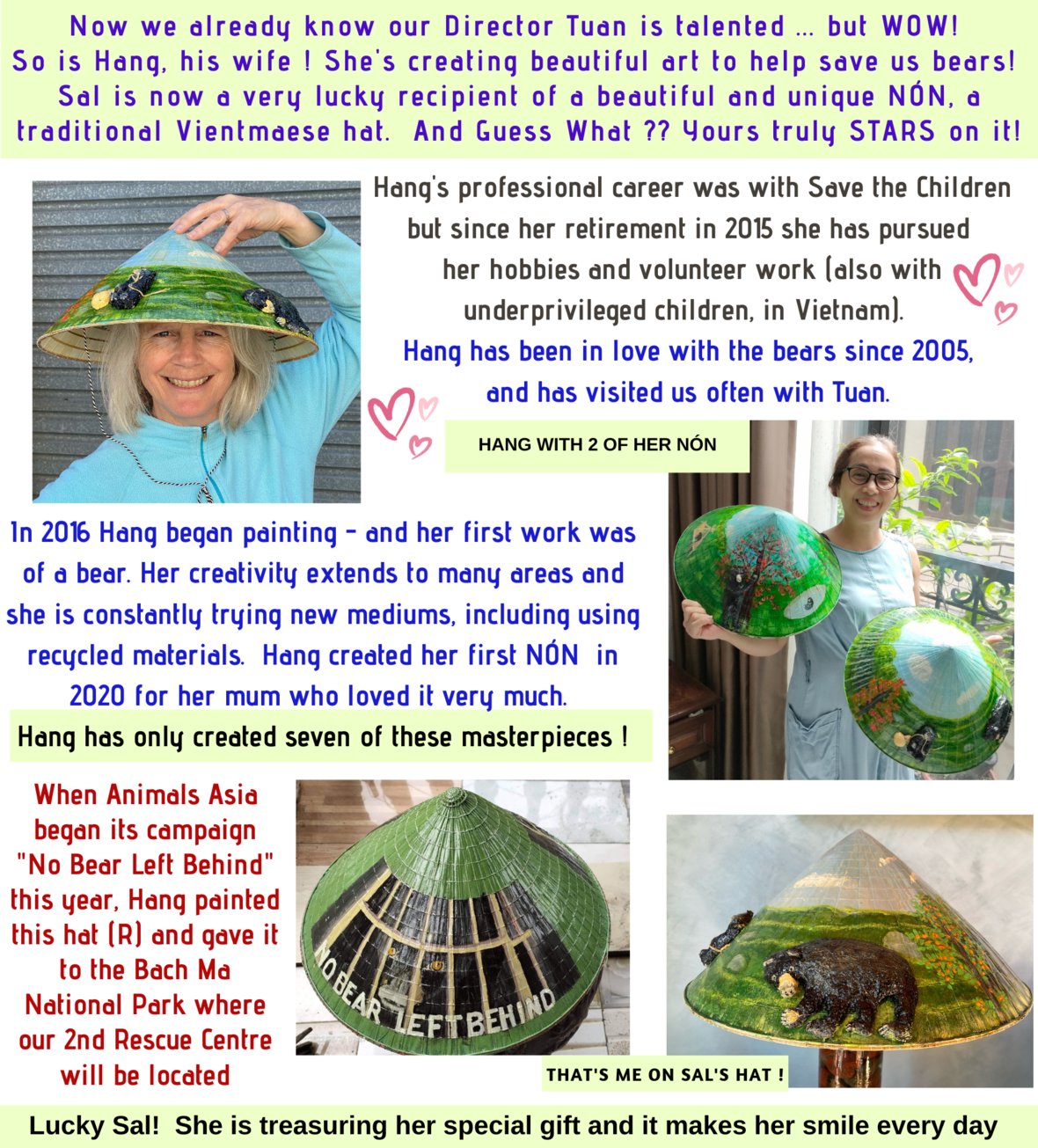table div table+table+table+table+table+table+table+table+table+table+table+table+table+table+table+table+table+table+table+table+table+table+table+table+table+table+table+table+table+table+table+table+table+table div table{width:100%;padding:0}table div table+table+table+table+table+table+table+table+table+table+table+table+table+table+table+table+table+table+table+table+table+table+table+table+table+table+table+table+table+table+table+table+table+table div table img{width:96.23%;padding:0;float:none}table div table+table+table+table+table+table+table+table+table+table+table+table+table+table+table+table+table+table+table+table+table+table+table+table+table+table+table+table+table+table+table+table+table+table div table td{width:100%;padding:0 1.88% 18px}/* styles */## Thanks for reading, everyone ! Looking forward to sharing more news from my bear world soon. Love, your bear Jigsaw! And remember .....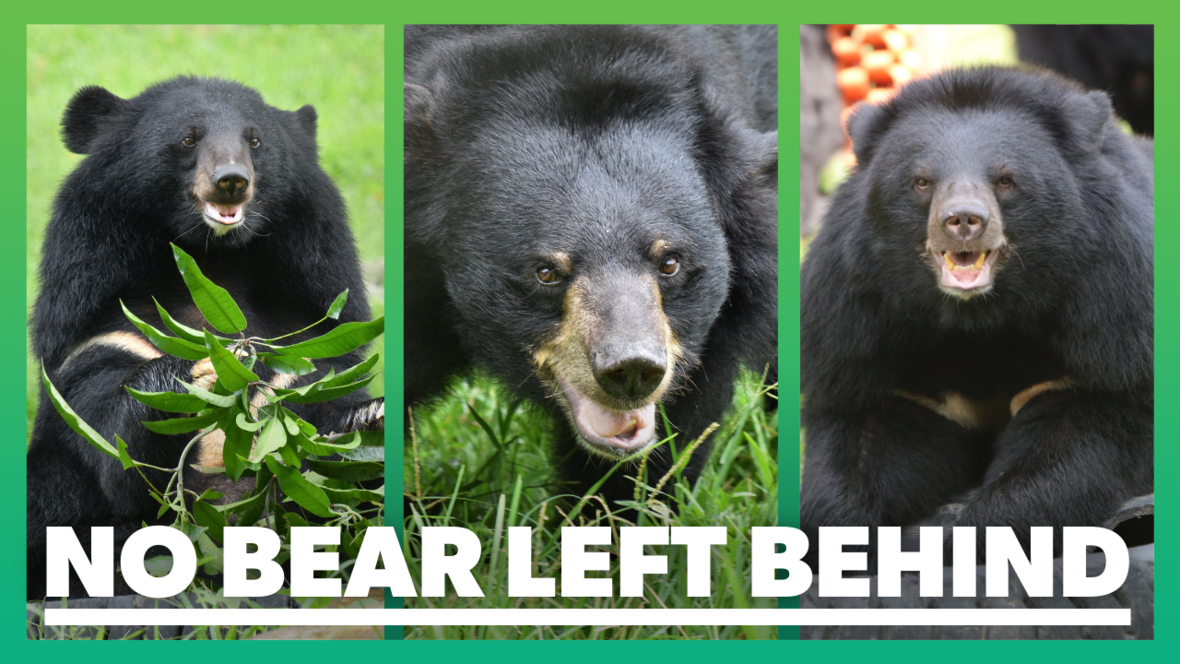TO FINISH: A BEAUTIFUL VIDEO OF TWO BEARS IN LOVE

## ps I know I promised you more news about our new sanctuary but I think I should stop now. I'll bring you a round up on Bach Ma in our December newsletter.

 table div table+table+table+table+table+table+table+table+table+table+table+table+table+table+table+table+table+table+table+table+table+table+table+table+table+table+table+table+table+table+table+table+table+table+table+table+table+table div table{width:100%;padding:0}table div table+table+table+table+table+table+table+table+table+table+table+table+table+table+table+table+table+table+table+table+table+table+table+table+table+table+table+table+table+table+table+table+table+table+table+table+table+table div table img{width:96.23%;padding:0;float:none}table div table+table+table+table+table+table+table+table+table+table+table+table+table+table+table+table+table+table+table+table+table+table+table+table+table+table+table+table+table+table+table+table+table+table+table+table+table+table div table td{width:100%;padding:0 1.88% 18px}/* styles */table.module-38{width:86.23%;padding:0}table div table+table+table+table+table+table+table+table+table+table+table+table+table+table+table+table+table+table+table+table+table+table+table+table+table+table+table+table+table+table+table+table+table+table+table+table+table+table+table div table{width:86.23%;float:none;margin-left:auto;margin-right:auto;padding:0}table div table+table+table+table+table+table+table+table+table+table+table+table+table+table+table+table+table+table+table+table+table+table+table+table+table+table+table+table+table+table+table+table+table+table+table+table+table+table+table div table a{border:0 none;text-decoration:none}table div table+table+table+table+table+table+table+table+table+table+table+table+table+table+table+table+table+table+table+table+table+table+table+table+table+table+table+table+table+table+table+table+table+table+table+table+table+table+table div table img{width:100%!important;border:0 none;text-decoration:none}table div table+table+table+table+table+table+table+table+table+table+table+table+table+table+table+table+table+table+table+table+table+table+table+table+table+table+table+table+table+table+table+table+table+table+table+table+table+table+table div table td{width:100%;padding:0}/* styles */
 table div table+table+table+table+table+table+table+table+table+table+table+table+table+table+table+table+table+table+table+table+table+table+table+table+table+table+table+table+table+table+table+table+table+table+table+table+table+table+table+table div table{width:100%;padding:0}table div table+table+table+table+table+table+table+table+table+table+table+table+table+table+table+table+table+table+table+table+table+table+table+table+table+table+table+table+table+table+table+table+table+table+table+table+table+table+table+table div table img{width:96.23%;padding:0;float:none}table div table+table+table+table+table+table+table+table+table+table+table+table+table+table+table+table+table+table+table+table+table+table+table+table+table+table+table+table+table+table+table+table+table+table+table+table+table+table+table+table div table td{width:100%;padding:0 1.88% 18px}/* styles */Like   Tweet   Pin   +1